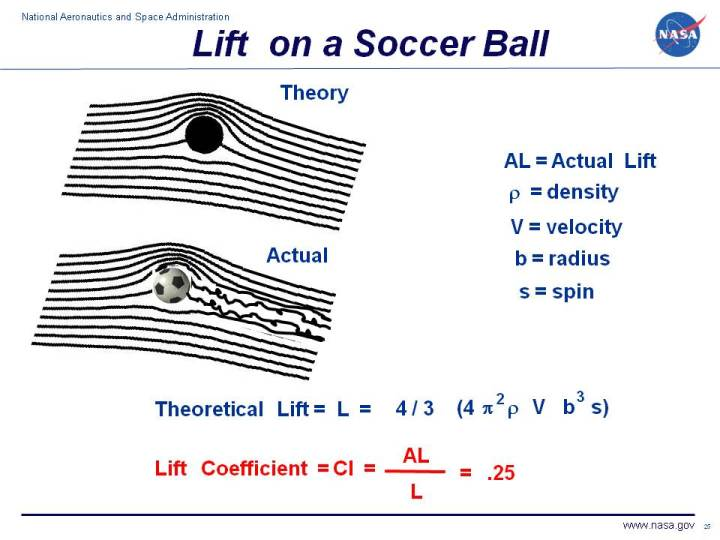+ Text Only Site
+ Non-Flash Version
+ Contact GlennAs an object moves through the air, the air is disturbed by the object. The disturbance generates an aerodynamic force on the object. Since a force is a vector quantity, having both a magnitude and a direction, we can split the aerodynamic force into two components. Drag acts in the direction of travel of the object. Lift acts perpendicular to the direction of travel. Many different objects are capable of generating a lift force. In particular, a spinning ball generates a force that is perpendicular to both the flow direction and to the axis of rotation. The lift force generated by a spinning soccer ball is the origin of the "bend", a curved kick to elude the goal keeper. For a "bend", the direction of the lift force is used to move the ball parallel to the surface of the earth, from side to side. The lift force on a soccer ball can also be used to loft or dive the ball. On this page we will discuss some of the details of the lift of a soccer ball. The details of how a moving soccer ball creates lift are fairly complex. If we move with an object through the air, the object appears to stand still and the air moves past the object at the speed of the object. Next to the surface of the object, the molecules of the air stick to the surface, as discussed in the properties of air slide. This thin layer of molecules entrains or pulls the surrounding flow of air. For a spinning ball the external flow is pulled in the direction of the spin. If the ball is not translating, we have a spinning, vortex-like flow set up around the spinning ball, neglecting three-dimensional and viscous effects in the outer flow. If the ball is translating through the air at some velocity, then on one side of the ball the entrained flow opposes the free stream flow, while on the other side of the ball, the entrained and free stream flows are in the same direction. Adding the components of velocity for the entrained flow to the free stream flow, on one side of the ball the net velocity is less than free stream; while on the other, the net velocity is greater than free stream. The flow is then turned by the spinning ball, and a force is generated. Because of the change to the velocity field, the pressure field is also altered around the ball. The magnitude of the force can be computed by integrating the surface pressure times the area around the ball. The direction of the force is perpendicular (at a right angle) to the flow direction and to the axis of rotation. On another page we develop the mathematical equation for the ideal theoretical lift of a spinning, smooth ball. L = (4 * pi^2 * b^3 * s * rho * V) * 4 / 3 where b is the radius of the ball, s is the spin of the ball, rho is the density of the air, V is the velocity of the ball, and pi is the standard 3.1415... The equation given above describes the theoretical lift force generated on a smooth, rotating ball. The ideal streamlines around a smooth ball are shown on the figure at the top left, marked "Theory". But in reality, the flow around a spinning soccer ball is very complex and looks more like the "Actual" flow shown on the figure. Viscosity generates a boundary layer on the ball and the stitches used to hold the covering of the ball together stick up out of the boundary layer and disturb both the boundary layer and free stream flow. On the ball, the boundary layer transitions to turbulent flow which affects the amount of aerodynamic force. The stitches are not symmetrically distributed around the ball. So the real flow around the ball is separated, unsteady, and not uniform. We can use the theoretical lift as a first order, or preliminary, estimate of the lift on a soccer ball. But to account for these real-world effects that we have neglected in our ideal flow model, we define a lift coefficient. The lift coefficient is an experimentally determined factor that is multiplied times the ideal lift value to produce a real lift value. The ideal simplified model tells us the relative importance of the factors that affect the lift force on the soccer ball, while all of the complex factors are modeled by the lift coefficient. Using experimental data for the movement of a kicked ball, Goff and Carre determined the average value of the lift coefficient to be about .25. We have used this value as the default value for the lift coefficient in the SoccerNASA simulation program You can further investigate the lift of a spinning soccer ball by changing the value of any of the factors that affect lift in the program. The lift force on the soccer ball is very similar to the lift generated by an airplane wing or a big league curve ball. Activities: Guided Tours Navigation ..NASA Soccer Home Page Beginner's Guide Home Page+ Inspector General Hotline + Equal Employment Opportunity Data Posted Pursuant to the No Fear Act + Budgets, Strategic Plans and Accountability Reports + Freedom of Information Act + The President's Management Agenda + NASA Privacy Statement, Disclaimer, and Accessibility CertificationEditor: Nancy Hall NASA Official: Nancy Hall Last Updated: May 05 2015 + Contact Glenn